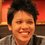# True or False questions?

I like how Brilliant have improved these past few months. I would like to add another feature called "True/False questions".

Segregating between true and false propositions allows users to understand the mechanics and avoid misconceptions and faulty generalizations. It also signifies a maturity of understanding of basics of math theory.

For example:

Q1) $\sum_{i=1}^\infty 1/n^{1.2}$ diverge. {+50, -100}

Q2) There are finite prime numbers. {+25, -50}

Q3) $x^2+x+123=0$ has no real solution. {+25, -75}

Q4) $\pi$ is irrational but not transcendental. {+100, -200}

Q5) $\sum_{n=2}^\infty (1 - \frac {1}{n^2} )^{n^2}$ diverges. {+125, -400}

Q6) $(1+2+ \ldots + n)$ divides $(1^3 + 2^3 + \ldots + n^3)$ for all natural numbers $n$. {+125, -400}

Q7) If $a_n \rightarrow 0$, then $\sum_{n=1}^\infty \frac {a_n}{a_n ^2 + n^2}$ converges absolutely. {+175, -1000}

Q8) For all positive integers $n$, integral of $(\sin x)^{n} dx$ from $0$ to $\pi /2$ is always a rational multiple of $\pi$. {+175, -900}

Q9) For all $0 , $(\sin x)^2 \leq \sin x^2$. {+180, -2000}

Q10) $4^{79} < 2^{100} + 3^{100} < 4^{80}$ is true. {+230, -3000}

If you answered correctly, you get points depending on the difficulty of the problem. You also get your points deducted if you answered wrongly. The simpler the question, the less points you're awarded or deducted, e.g {+100, -100}. However, the harder the problem, there's slight increment of points you're awarded but a much higher deduction of points when you're wrong, e.g {+200, -2500}

Users are not allowed to solve the problem if they don't have sufficient points to be deducted.Note by Pi Han Goh
6 years, 9 months ago

This discussion board is a place to discuss our Daily Challenges and the math and science related to those challenges. Explanations are more than just a solution — they should explain the steps and thinking strategies that you used to obtain the solution. Comments should further the discussion of math and science.

When posting on Brilliant:

• Use the emojis to react to an explanation, whether you're congratulating a job well done , or just really confused .
• Ask specific questions about the challenge or the steps in somebody's explanation. Well-posed questions can add a lot to the discussion, but posting "I don't understand!" doesn't help anyone.
• Try to contribute something new to the discussion, whether it is an extension, generalization or other idea related to the challenge.

MarkdownAppears as
*italics* or _italics_ italics
**bold** or __bold__ bold
- bulleted- list
• bulleted
• list
1. numbered2. list
1. numbered
2. list
Note: you must add a full line of space before and after lists for them to show up correctly
paragraph 1paragraph 2

paragraph 1

paragraph 2

[example link](https://brilliant.org)example link
> This is a quote
This is a quote
    # I indented these lines
# 4 spaces, and now they show
# up as a code block.

print "hello world"
# I indented these lines
# 4 spaces, and now they show
# up as a code block.

print "hello world"
MathAppears as
Remember to wrap math in $$ ... $$ or $ ... $ to ensure proper formatting.
2 \times 3 $2 \times 3$
2^{34} $2^{34}$
a_{i-1} $a_{i-1}$
\frac{2}{3} $\frac{2}{3}$
\sqrt{2} $\sqrt{2}$
\sum_{i=1}^3 $\sum_{i=1}^3$
\sin \theta $\sin \theta$
\boxed{123} $\boxed{123}$

Sort by:

I guess one possible complaint is that the system is a lot more cheat-able. I mean, there are only two possible answer choices, in contrast to the 1000 possible answer choices of our current problems. As such, even if you get it wrong, you know what the right answer is and can tell someone else, whereas with the current system even if you get the problem wrong 3 times you still might not know the answer.

However, I do think this would be pretty nice implemented into practice, because it's also a lot easier for people to analyze where they went wrong.

- 6 years, 9 months ago

Brilliant has stated that problems which use straight-forward theorems and formulas are not going to be shown on Brilliant's feature problem. And yes, some users can tell others the answer.

- 6 years, 9 months ago

The first eight questions are pretty straightforward, the last two ain't that straightforward. And somemore, the staff can always change the question. like $x^2+x+123=0$ to $x^2+x-1=0$

- 6 years, 9 months ago

I'm not sure if I would attempt these problems with these win/loss ratios.

- 6 years, 9 months ago

Why not?

- 6 years, 9 months ago

I wouldn't risk losing thousands of points if the good answer only gives me a few hundred.

- 6 years, 9 months ago

I didn't literally mean that if you're wrong, you lose thousands of points, I'm just saying you will lose more than you gain if you're wrong. I don't exactly know how much points we should deduct if we're wrong. This is just a concept. If you're right, you get points as usual; if you're wrong, your points are deducted. Still don't like it?

- 6 years, 9 months ago